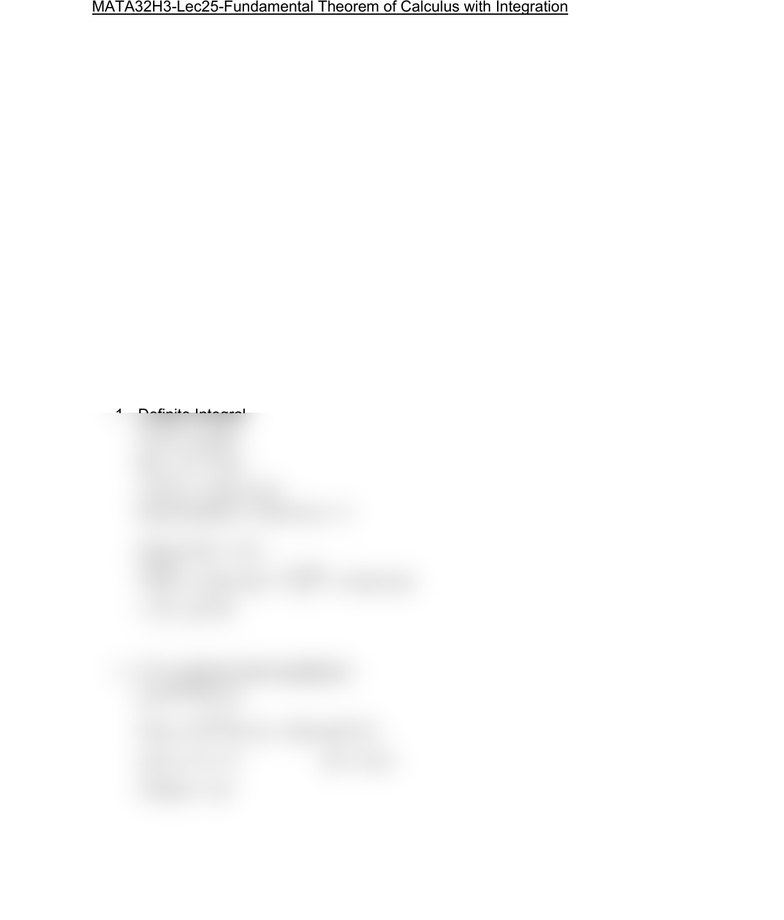Class Notes (1,100,000)
CA (640,000)
UTSC (30,000)
Mathematics (1,000)
MATA32H3 (200)
Lecture 25

# MATA32H3 Lecture Notes - Lecture 25: AntiderivativePremium

Department
Mathematics
Course Code
MATA32H3
Professor
Karimian Pour, C.
Lecture
25

This preview shows half of the first page. to view the full 2 pages of the document.MATA32H3-Lec25-Fundamental Theorem of Calculus with Integration
If f(x) > 0 if and only if x equals real numbers on [a,b] then

= { area of the region beneath y=f(x) where a ≤ x ≤ b .
Fundamental Theorem of Calculus (FTC)
If y=f(x) is a continuous function on [a,b] and F is any antiderivative then

= F(b) F(a)
Easy form
Substitution
Integration by parts
*Once you have F(x) = 
Examples:
1. Definite Integral

f(x) = + 1/4x
 + (1/4)

(((2x)^(3/2))/3) + (1/4) ln (x) + C
Plug in F(4) F(1)
+ (1/4) ln (4) -
+ (1/4) ln (4)

+
ln (4)
2. FTC combined with substitution

Find  , then use FTC
Let u = x^2 + 6 du = 2x dx
(1/2)du = x dx